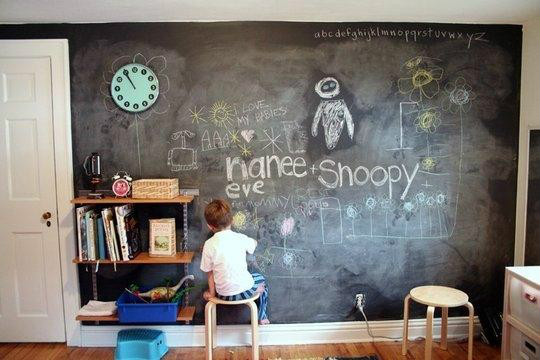# VIRTUAL MANIPULATIVES

11/24/2015 by in category INDUSTRIAL NEWS with 0 and 1
Home > Blog > INDUSTRIAL NEWS > VIRTUAL MANIPULATIVES### VIRTUAL MANIPULATIVES

Manipulatives (blocks, rods, bean sticks, etc.) are commonly used in mathematics education.

In recent years, a number of websites have been developed that contain “virtual” versions of some of these manipulatives. The references given below provide information about some of these websites.

It appears that there may be a fine dividing line between a concrete manipulative and a virtual manipulative. For example, does it make any difference in student learning whether a physical spinner is used to generate data, or whether a computerized spinner (that may well look the same) is used?

One of the most important general developments in science in recent years has been the idea of Computational Science. Thus, we now have Computational Biology, Computational Chemistry, etc. (The same idea applies to other disciplines, and these other disciplines have had varying levels of success in incorporating IT within their basic fabric.) And, as might be expected, Computational Mathematics is now an important branch of mathematics.

The essence of Computational “XXXX” (name a discipline) is the development and use of mathematical models that can be implemented on a computer. Thus we have 1) The “real thing.”; The mathematical model.; and 3) The Computational Model (simulation). This has deep educational ramifications, both in math education and in each other discipline. In brief summary:

Math education needs to include both math modeling and computer implementation of math models (computer simulations). This needs to be taught/learned in a manner that transfers to disciplines outside of math.

Each discipline needs to be examined from the point of view of the math modeling and computer simulation that has become and/or is becoming an integrated component of the discipline. Thus, each teacher of a specific discipline needs to help students learn current and potential roles of math modeling and computer simulation to represent and help solve the problems of the discipline.

As an example, suppose that a math teacher is teaching students to use a spreadsheet. The spreadsheet is an excellent aid to developing math models of certain types of business problem situations. And, of course, spreadsheet models/simulations are used in many other disciplines. Thus, the math teacher is responsible both for teaching use of the spreadsheet to develop and implement models, but also how to use this tool in a variety of areas such as business, social studies, and so on. The business teacher, the social students teacher, and so on have the responsibility both of having students develop an appropriate level of fluency in modeling/simulation within their disciplines, but also carrying students to greater depths in the types of problems that are addressed by this approach.

In summary, when a math teacher has children use a virtual manipulative, the math teacher has the opportunity to help students learn some of the underlying ideas of math modeling and computer simulation. This is quite a different goal than merely using the virtual manipulative as a substitute (be it inferior of superior) the the real concrete manipulative that it models.